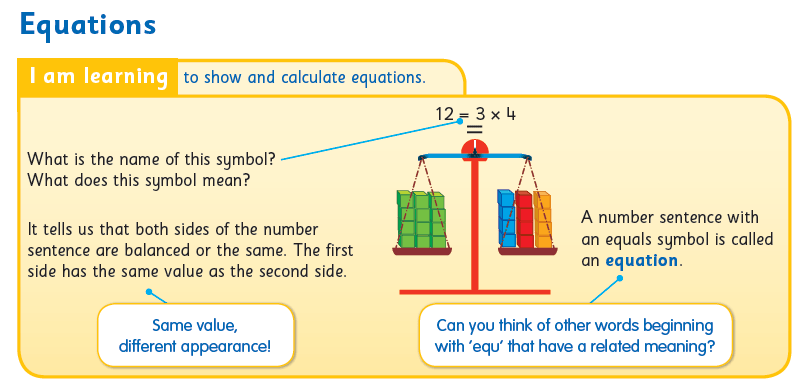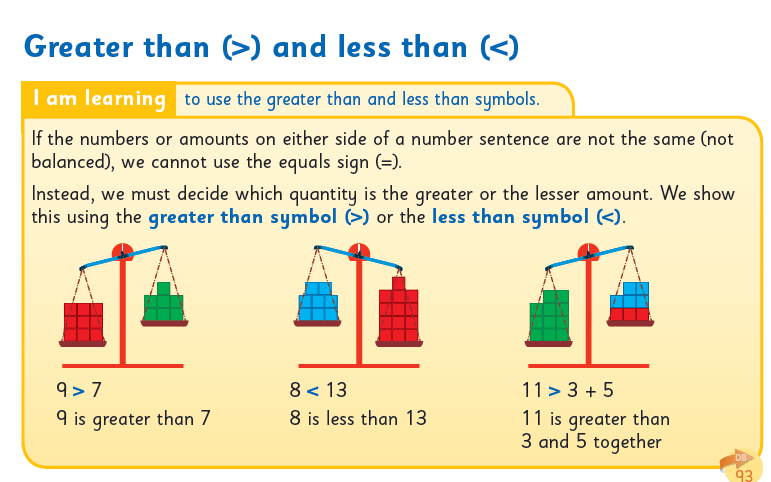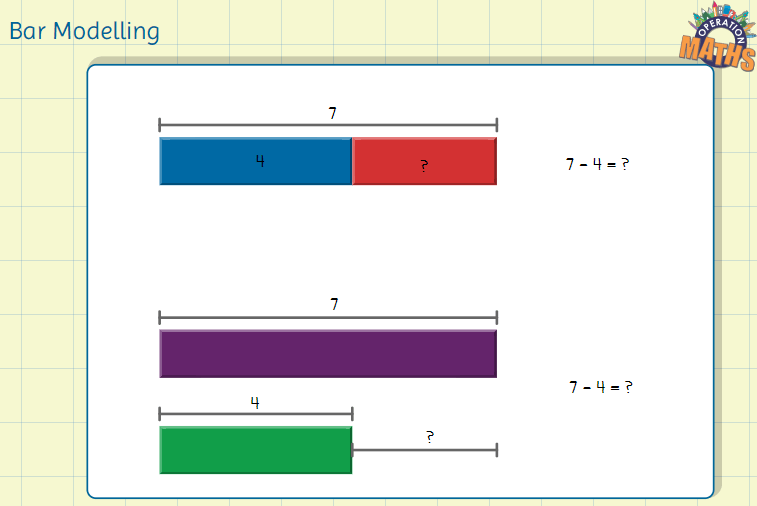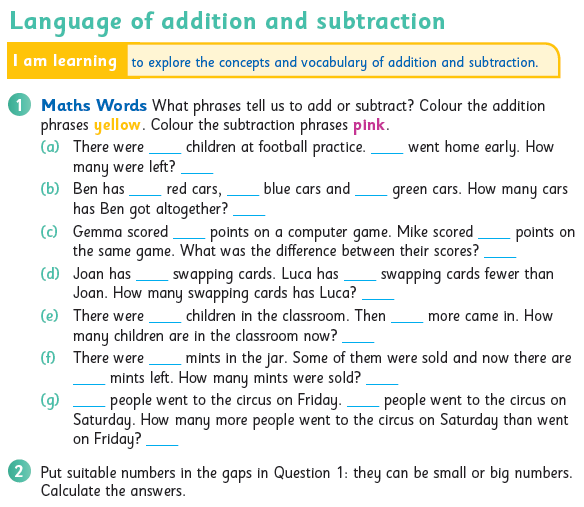# Digging Deeper into … Number Sentences, Equations & Variables (3rd – 6th)

## Digging Deeper into … Number Sentences, Equations & Variables (3rd – 6th)

Category : Uncategorized

In the Primary Mathematics Curriculum (1999), this topic appears as three separate strand units, all within the strand of Algebra:

• Number Sentences (3rd & 4th class)
• Equations (5th & 6th class)
• Variables (6th class)

However, since these concepts are intrinsically connected, in Operation Maths they are taught in a cohesive and progressive way through third to sixth class.

• Number sentences are mathematical sentences written using numerals (e.g. 1, 5, 67, 809, 1.45, 1/2  etc.) and mathematical symbols (e.g. +, -, x, ÷, <, >, =).
• They include both equations (see below) and inequalities (64 < 82, 23 > -16), although the term inequalities is not specifically used.
• The unknown or missing value in a number sentence (i.e. a variable) can be represented by a frame (box), by a shape, or by a letter, although it should be noted that the Primary Mathematics Curriculum (1999) specifies a preference for a frame (box), up to the introduction of variables in 6th class
• An Equation is a special type of number sentence, containing an equals sign, to show that two expressions are equal (e.g. 5 = 3 + 2, 5 + 6 = 20 – 9, etc.)
• A variable is a value in an expression that can  change or vary. However, when there is only one variable in an equation then the value of that variable can be calculated e.g. a + 6 = 9, 20 = 4b.

Thus, while these strand units are only being formally introduced from third class on, the children have actually been exposed to number sentences, equations and variables (i.e. the frame) since the infant classes.

### Equations

(aka Number sentences with an equals sign)

Understanding equations necessitate the appreciation of the correct meaning of the equals symbol. Many children incorrectly translate the equals symbol (=) as meaning ‘and the answer is…’. This incorrectly reinforces that both its purpose and position is to precede the answer in any calculation, a misconception also reinforced by calculators, where you press the = button to get the answer. Such misunderstanding is
evident when you see responses like these:
5 + 6 =  + 3 , i.e. ‘5 + 6 is 11’
5 + 6 =  + 3 , i.e. ‘5 + 6 + 3 is 14’
Adults may also unwittingly compound this, by using ‘makes’ or ‘gives’ as a synonym for equals.

It is vital that the children recognise that the equals symbol indicates that both sides of the equation (which will be referred to simply as a number sentence until fifth class, when the term “equation” is introduced), are equal to one another/are the same value/are balanced. In this way an actual balance (pan or bucket) and cubes can be extremely valuable to model (and solve) equations e.g. in the images below, the first balance shows that 5 equals a group of 3 and a group of 2, and the second balance shows that 12 equals 3 groups of 4.From Operation Maths 4From Operation Maths 5

Furthermore, teachers should reinforce the correct meaning for the symbol = by only translating it as ‘equals’, ‘is equal to’ and/or ‘is the same as’.

### Inequalities

(aka Number sentences with greater than/less than sign)

Despite the fact that the children have been using the greater than and less than symbols since 2nd Class, many still have difficulties reading them and interpreting their meaning. Using a balance and concrete materials, in a similar way as when teaching equations, can greatly help children to gain deeper understanding of the symbols and their meanings.From Operation Maths 4

Through exploration they can identify what is the maximum number of cubes they can put on a side that is less than the other side, before it makes the balance tip in the other direction, thereby invalidating the number sentence; or the minimum number of cubes they can put on a side that is greater than the opposite side, so as to keep the number sentence true.

### Using estimation strategies

Often, when having to indicate if a given number sentence is true or false, it is not always necessary for the children to calculate both sides of the number sentence exactly. There is (usually) only one true or correct option, meaning that every other answer is incorrect or false. Encourage the children to use their estimation and number sense skills to quickly recognise when a statement is obviously false, e.g. a big difference in the size of numbers on one side versus the other.While some might view this as a type of ‘cheat’ strategy, in truth, it is more about identifying the most efficient approach, while also reinforcing the value of estimation in general and, particularly, as a way to make calculations easier.

### Translating number sentences into word problems and vice-versa

As mentioned earlier, this is in fact a skill that the children would have been exposed to, and been using, since the infant classes. Furthermore, as this topic is deliberately positioned towards the end of the yearly plans in Operation Maths 3-6, the children will have already been using this skill very regularly in the number, data and measures chapters, prior to this point of the school year.

The curriculum specifies that the children should be enabled to translate number sentences into word problems, both of which can be viewed as abstract representations. Worth noting, is that the curriculum doesn’t emphasise the importance of the translating the number sentences and word problems into concrete and/or pictorial representations. Whereas, in Operation Maths, (in keeping with its overarching CPA approach) , there is significant emphasis placed also on utilising various concrete materials and visual strategies to represent the word problems and number sentences.From Operation Maths 5

The development of visual strategies for problem-solving,  is a central focus of the work throughout the Number chapters. Thus, this topic allows the teacher to revise the visual strategies covered so far and assess how well the children understand them and can apply them.

The interconnectedness of real-life scenarios and mathematical sentences/equations should also be emphasised. At primary level, there should always be some relatable context for any number sentence.
For many children, when looking at a number sentence, it can be difficult to appreciate how a collection of digits and symbols could relate to a real-life scenario with which they can identify. That is essentially what a word problem is; it provides a real-life context within which to frame the numbers and operators involved. Emphasise to the children throughout this topic how the number sentences could be given a real-life story (i.e. word problem), and encourage them to come up with possible stories either verbally or written down.

And, depending on the context given to a particular story, the visual representation may also be different, even though the number sentence/equation may stay the same. For example for the number sentence 7 – 4 = ? the word problem (context) could be either of the following:

• Áine has 7 cookies. Abdul has 4 cookies. How many more cookies has Áine than Abdul?Image created using Bar Modelling eManipulative, accessible on edcolearning.ie

And while the number sentences are the same, both the contexts and the pictorial representations (e.g. bar models, as shown above) are different, as they represent different types of subtraction. In the case of the first word problem, this is describing subtraction as deduction, and a part-whole bar model is more suitable. In the case of the second word problem, this is describing subtraction as difference, and a comparison bar model is more suitable.

### Identifying operation phrases

When the children are translating word problems into number sentences, it is very important that they can understand the context being described and are able to identify that phrases that indicate the operation(s) required.

Regularly interspersed throughout the operations chapters in the Discovery books for Operation Maths 3-6,  there are activities which enable the children to identify and colour-code the specific vocabulary that an indicate the required operation (see example below). This topic provides an ideal opportunity to review this skill and assess/re-teach the children accordingly.From Operation Maths 4, Discovery Book

In particular, many of the Talk Time activities, require the children to suggest ways to verbalise the various equations, e.g:

• The difference between 46 and 18 is equal to the product of 4 and 7; true or false?
• 18 subtracted from 46 equals 4 times 7; true or false?

Where possible the children should suggest alternative phrases for the same equation thus reinforcing the use of correct mathematical language.

### Input and Outputs

In Operation Maths 4 & 5, activities based on inputs and outputs are included as a means to consolidate the children’s understanding of number sentences and their ability to write number sentences (see below).  Input-output activities can provide great scope for problem solving, as well as preparing the children for calculations involving variables in sixth class.From Operation Maths 4

### Variables

Variables are formally introduced in sixth class, although the children have encountered variables (as a symbol or shape to represent a missing value) since they first encountered the frame (answer box).

When calculating with variables, both part-whole bar models and comparison bar models can be very useful to represent the relationship between the known and unknown values.From Operation Maths 6